#### Chapter 14 Quadrilaterals R.D. Sharma Solutions for Class 9th Exercise 14.3

Exercise 14.3

1. In a parallelogram ABCD, determine the sum of angles ∠C and ∠D.

Solution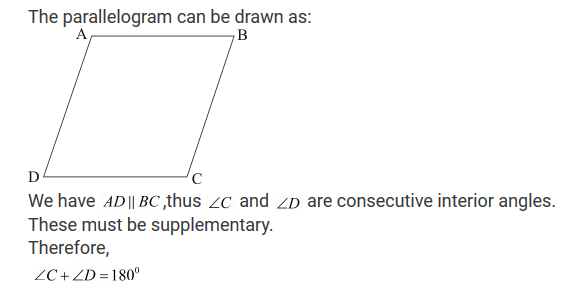2. In a parallelogram ABCD, if ∠B = 135°, determine the measure of its other angles.

Solution3. ABCD is a square. AC and BD intersect at O. State the measure of ∠AOB.

Solution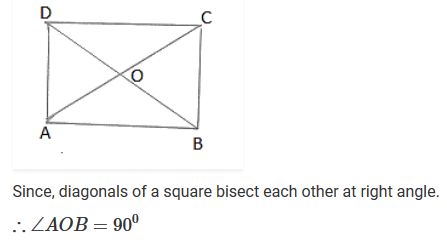4. ABCD is a rectangle with ∠ABD = 40°. Determine ∠DBC.

Solution5. The sides AB and CD of a parallelogram ABCD are bisected at E and F. Prove that EBFD is a parallelogram.

Solution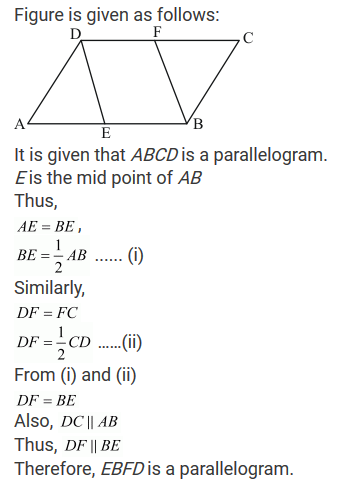6. P and Q are the points of trisection of the diagonal BD of a parallelogram ABCD. Prove that CQ is parallel to AP. Prove also that AC bisects PQ.

Solution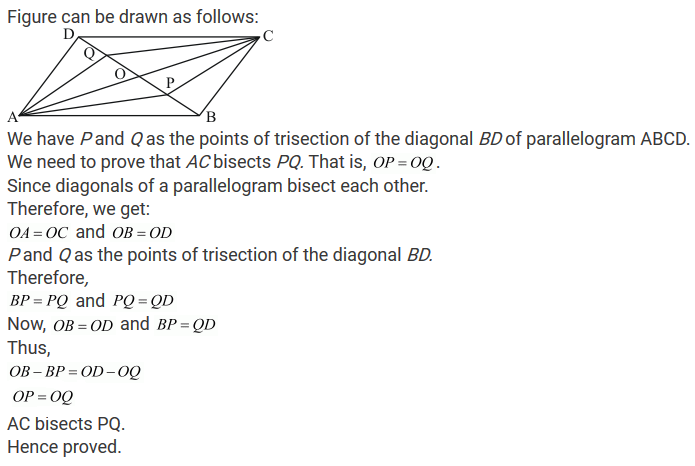7. ABCD is a square E, F, G and H are points on AB, BC, CD and DA respectively. such that AE = BF = CG = DH. Prove that EFGH is a square.

Solution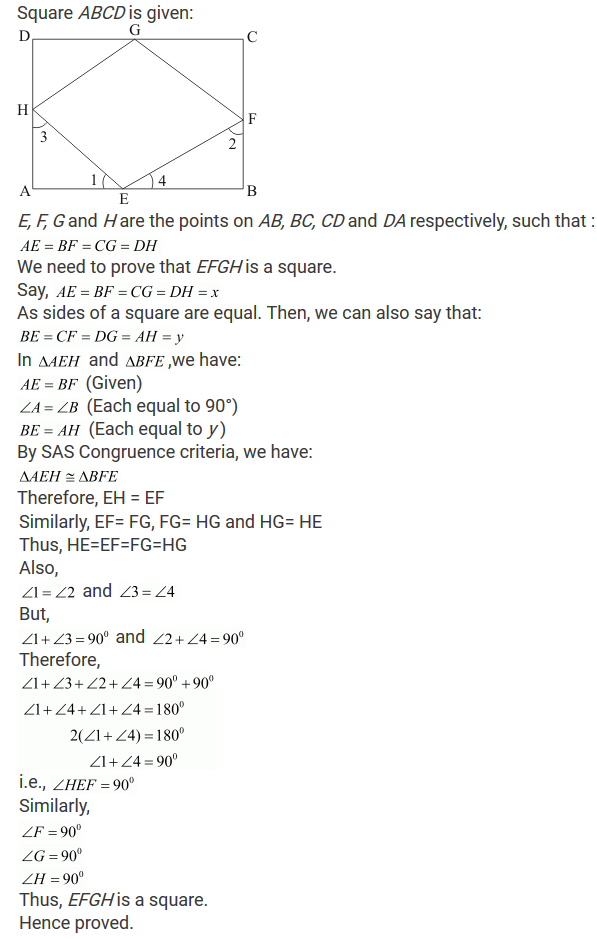8. ABCD is a rhombus, EABF is a straight line such that EA = AB = BF. Prove that ED and FC when produced meet at right angles.

Solution9. ABCD is a parallelogram, AD is produced to E so that DE = DC and EC produced meets AB produced in F. Prove that BF = BC

Solution